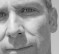## Goertzel filter with decay?Started by 8 years ago9 replieslatest reply 7 years ago459 views

Hi there

I'm trying to recreate a high-Q, second-order band pass filter that was popularized in the 1990s in the GRM Tools Reson plug-in. I recently discovered the #Goertzel algorithm which can be used as a single-frequency band pass filter, and it seems like exactly what I'm after. The trouble is that the Goertzel output (a sinusoid) doesn't decay. Unfortunately my DSP chops are not quite good enough to understand how I could modify the filter coefficients to create varying degrees of exponential decay. I'd be happy for some pointers on how I might do this, or indeed if there are any other single-frequency resonator algorithms I should be investigating.

[ - ]I'll try not to rant about the Goertzel.  It's a perfectly good algorithm, except that it is really a special case of a resonator with infinite Q.

You want a nice sharp band-bass filter, but one with a finite Q.

I'm going to digress into z-domain notation here -- I know that this is probably beyond what you're used to dealing with, but this article may help: <http://wescottdesign.com/articles/zTransform/z-tra...>.  The "pure" Goertzel isn't really a filter per se, but it's various bits have responses of the form

H_g(z) = -------------------

z^2 - cos(th)*z + 1

To get a bandpass filter with a non-infinite Q, you need a transfer function of the form

k * (z^2 - 1)

H_bp(z) = ---------------------------

z^2 - d * cos(th) * z + d^2

Aaagh (June 14, 2016)!  Screwed that one up!  I should have written:

$$H_{bp} \left ( z \right ) = \frac {k \left ( z^2 - 1 \right )}{z^2 - 2 d \cos (\theta) z + d^2}$$

Sorry 'bout that.

(There are other 2nd-order bandpass filters out there -- this is just the simplest).

Setting d < 1 will give you damping.  The advance angle, th, is roughly the peak of the filter response in radians/sample (which you convert to Hz by knowing your sample rate, and knowing that there are 2 * pi radians in a cycle).

Realize this with a biquad filter.  You'll have some interesting adventures if you have a really high Q, because precision (and overflow, if you use fixed-point math) will be problems.

If this isn't enough to get you started, ask more.

[ - ]Are we dealing with integers or floating variables? Shouldn't we drop the Least Significant Bit to avoid oscillation -- if you slide this algorithm over too many samples?

[ - ]Thanks so much for that. I'm a bit rough in my conversions of transfer functions to direct form, but am I right in thinking that would convert to the following?

y[n] = k*x[n]-k*x[n-2]+d*cos(th)*y[n-1]-d^2*y[n-2]

Also, what does the term 'k' represent?

[ - ]Yes, but please see my correction to my February post.

'k' is just a gain, so that the peak gain of the filter is something manageable.  Trying to find a general expression for it is a pain in the behind, but if you're working at a fixed frequency it's pretty easy to solve for the gain where 'k' is 1 and then correct as you will.

[ - ]Let me ping a couple of gurus in case they can/want to help: @Tim Wescott, @Rick Lyons, @Slartibartfast

[ - ]Hi Michael,

being quite new to dsp, I just happen to have stumbled over Goertzel like you. As I have learned for my application: I get a sequence of samples - just as if I were to do a DFT, eventually (e.g. if there is noise) apply a window function (e.g. Hamming) and then run through Goertzel's algorithm to end up with a real and imaginary component (or, alternately amplitude and phase) for the intended frequency as contained in my samples. For all this action, the signal I want to detect or analyze can be considered as stationary.

Now, if you expect a change of amplitude (e.g. decay) just repeat the process over and over and check amplitude. For an output of the "filtered" signal just emit a sine of like amplitude and phase.

I hope I have understood your needs and been helpful - Klaus

[ - ]Hi everyone — thanks for your input.

I modified the Goertzel algorithm by adding the following decay terms to the coefficients (which I think makes it a standard biquad as per Tim's reply above):

theta = 2*M_PI*f->frequency/x->DSPSampleRate;

a0 = 0.1; // gain term

a1 = decay * 2*cosf(theta);

a2 = decay * decay;

b1 = cosf(theta);

sn2 = previoussn;

sn1 = previoussn;

sn = a0*input + a1*sn1 - a2*sn2;

output = sn - b1*sn1 - a0*input;

It works now, but it still seems to let quite a bit of the 'original sound' through, which suggests that the filter stopband is not attenuating enough.

Have a listen to the GRM Reson filters, and how they've managed to almost completely attenuate the sound outside of the passband, despite their documentation claiming it's just a 'second-order filter':

Looking at the impulse response of this filter (see image) it seems to have about a -48dB per octave skirt, which is pretty steep — any thoughts on how that might be achieved with 'just' a second-order filter? Perhaps an elliptic filter?[ - ]